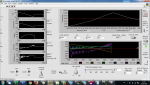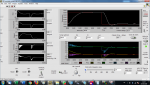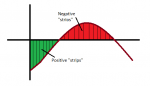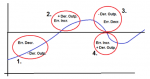# Microcontroller based PID controller

Hi all,
This is my firs post in this forum.
I design simple PID controller (programing Microchip PIC18F2550) based on theory I have learned from Control system on college. I am newbie in PID control, so I am sorry if I ask something what is simple.

I would like whether my controller was designed correctly.
Description:
Heater element 24V 40W is in the aluminum heat sink with blades (size 6cm x 6cm x 1cm block plus 5 blades height 3cm). The temperature is controlled by micro-controller (the PID controller is programmed in the micro-controller) by 19.5V PWM (power source used from old laptop).

Output of the controller is sum of P-control, I-control and D-control. The sign of the output signal is given by the sum of those three control signals. (positive sign = heater is ON, negative sign = heater is OFF - heat sink is cooled by ambient air ). The output (sum of P-control, I-control and D-control) is fed in to PWM 10bit register. So value 0 = 0%PWM Duty cucle and 1023 = 100%PWM Duty cycle. For instance if PID controller output is 767, then PWM Duty cycle is set o 75%. If PID output is 1500 (it is more than 1023) then PWM regisr is set to 1023 (100% PWM).
Ths is how I set the realtion beteen the output signal and power signal for heater element.
Q1: Is that way right or it should be done different way?

The PID controller looks works fine, but I have one problem and I do not know whether it is normal for PID controller or not.
After many tuning tests, I have find out that if I tune the PID controller to reach e.g. 80°C from ambient temperature (system is turned ON) the setpoint is reached with no or very small overshoot, but it regulates the temperature around set-point (+5°C -5°C) slowly - response is slow.

If I tune the PID controller to control the temperature relatively fast around the set-point (+5°C -5°C), regulation works fine around setpoint Picture 2, but at system start up the temperature raise very high over 80°C (up to 120°C and it was still raising even the integral output has started to decrease Picture 1) due to very high integral control output value (area from 20°C up to 80°C is huge what causes that Integral value build up at 80°C up to around 1000 (97%PWM), so heater power 97%.

Picture 1Picture 2So there are two options for controller tuning
a) Controller is tuned to reach 80°C from ambient temperature with very tiny overshoot
or
b) Controller is tuned to control the temperature around 80°C

Is this behaviour normal for PID controller or not and my program code is incorrect?

This is the way how I calculate the output of my PID controller:
Error
Error = Set-point - Current temp. (Error is positive if the measured temperature is below the set-point and negative if it is above the set-point)

Proprotional
Proportional output = Error * Kp
(Prop. output sign depend on error sign)

Integral
Integral output = Accumulated integral "strips" * Ki
(where Ki is integral parameter entered by user)
where
Accumulated integral "strips" = Accumulated integral "strips" + Actual integral strip
Actual integral "strip" = ( (Measured current error - Previous error)/2 ) * T (T= sampling period = 2 seconds), (sign of one integral "strip" depend on Error sign)
Picture 3Derivative
Derivative output = ( (Measured current error - Previous error) / T )* Kd
(T= sampling period = 2 seconds)
The sign of the derivative output is:
1. tempereture bellow setpoint and falling toward setpoint (error decrease) - negative
2. tempereture above setpoint and raising from setpoint (error inrease)- positive
3. tempereture above setpoint and falling toward setpoint (error inrease)- negative
4. tempereture bellow setpoint and raising from setpoint (error inrease)- positive
Picture 4Q2: Is this PID controller design correct?

Many thanks for help.

#### Dave

where you have long "dead times" and a single sensor, proportional only is the easiest to work with and doesn't over drive the system.